3

Split BST

Difficulty: MEDIUM
Avg. time to solve
25 min
Success Rate
75%

Problem Statement

Note:

1. A binary search tree is a binary tree data structure, with the following properties
a. The left subtree of any node contains nodes with the value less than the node’s value.
b. The right subtree of any node contains nodes with the value equal to or greater than the node’s value.
c. Right, and left subtrees are also binary search trees.
2. It is guaranteed that all nodes in the given tree will have distinct positive integral values .
3. The given tree may or may not contain a node of value equal to the given value ‘K’.

Example, below the tree, is a binary search tree.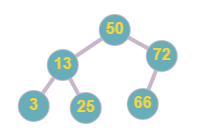Below the tree is not a BST as node ‘2’ is less than node ‘3’ but ‘2’ is the right child of ‘3’, and node ‘6’ is greater than node ‘5’ but it is in the left subtree of node ‘5’.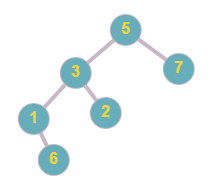Note:

1. You have to split the given tree in such a way that the structure of both returned trees is similar to the originally given tree, i.e. if a parent node ‘P’ and child node ‘C’ lies on the same tree after splitting, then ‘C must be the same child of ‘P’.
2. If there is no valid tree, then return ‘NULL’ node in its place.

Input Format:

The first line contains an integer 'T' which denotes the number of test cases or queries to be run.

The first line of each test case contains elements of the tree in the level order form. The line consists of values of nodes separated by a single space. In case a node is null, we take -1 in its place.

The second line of each test case contains a positive integer value ‘K’, denoting the value for splitting the given tree.

For example, the level order input for the tree depicted in the below image.50
13 72
3 25 66 -1
-1 -1 -1 -1 -1 -1

Explanation :

Level 1 :
The root node of the tree is 50

Level 2 :
Left child of 50 = 13
Right child of 50 = 72

Level 3 :
Left child of 13 = 3
Right child of 13 = 25
Left child of 72 = 66
Right child of 72 =  ‘Null’

Level 4 :
All children are ‘Null’

The first not-null node(of the previous level) is treated as the parent of the first two nodes of the current level. The second not-null node (of the previous level) is treated as the parent node for the next two nodes of the current level and so on.
The input ends when all nodes at the last level are null(-1).

Note :

The above format was just to provide clarity on how the input is formed for a given tree.
The sequence will be put together in a single line separated by a single space. Hence, for the above-depicted tree, the input will be given as:
50 13 72 3 25 66 -1 -1 -1 -1 -1 -1 -1

Output Format:

For each test case, the output will be “1” if you have returned the correct answer, else it will be “0”.
The output of each test case will be printed in a separate line.

Note :

You do not need to input or print anything, and it has already been taken care of. Just implement the given function.

Constraints:

1 <= T <= 5
1 <= N <= 3000
1 <= data <= 10 ^ 9
1 <= K <= 10 ^ 9
Where ‘T’ denotes the number of test cases, ‘N’ is the total number of nodes in the given binary tree, ‘data’ is the value of the nodes of the given binary tree, and ‘K’ represents the given value for splitting the tree.

For a single test case, all given ‘data’ are distinct from each other.

Sample Input 1:

2
7 5 10 3 6 8 11 2 -1 -1 -1 -1 -1 -1 -1 -1 -1
5
5 4 6 -1 -1 -1 -1
3

1
1

Explanation of Sample Input 1:

For the first test case, the given tree is.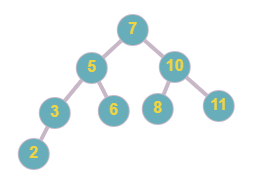All nodes less than or equal to ‘5’ will be part of the first tree and all remaining nodes will be part of the second tree. The tree after the split will look like the one below.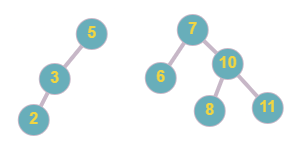The given tree in the second test case is -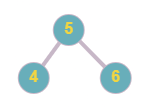All nodes in the tree are greater than the given value, thus we will return ‘NULL’ node in the first tree and the original tree as the second tree.

Sample Input 2:

1
10 2 12 1 3 -1 13 -1 -1 -1 -1 -1 -1
11

1

Explanation of Sample Input 2:

For the first test case, the given tree is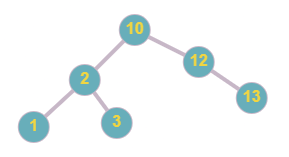The output trees are below.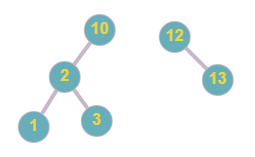Note that the below output tree is not correct because the structure is not maintained as node ‘12’ and ‘13’ lies on the same tree and ‘12’ is a child of ‘13’ in the output tree, but in the original tree ‘13’ is a child of ‘12’.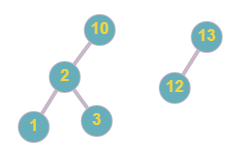Console